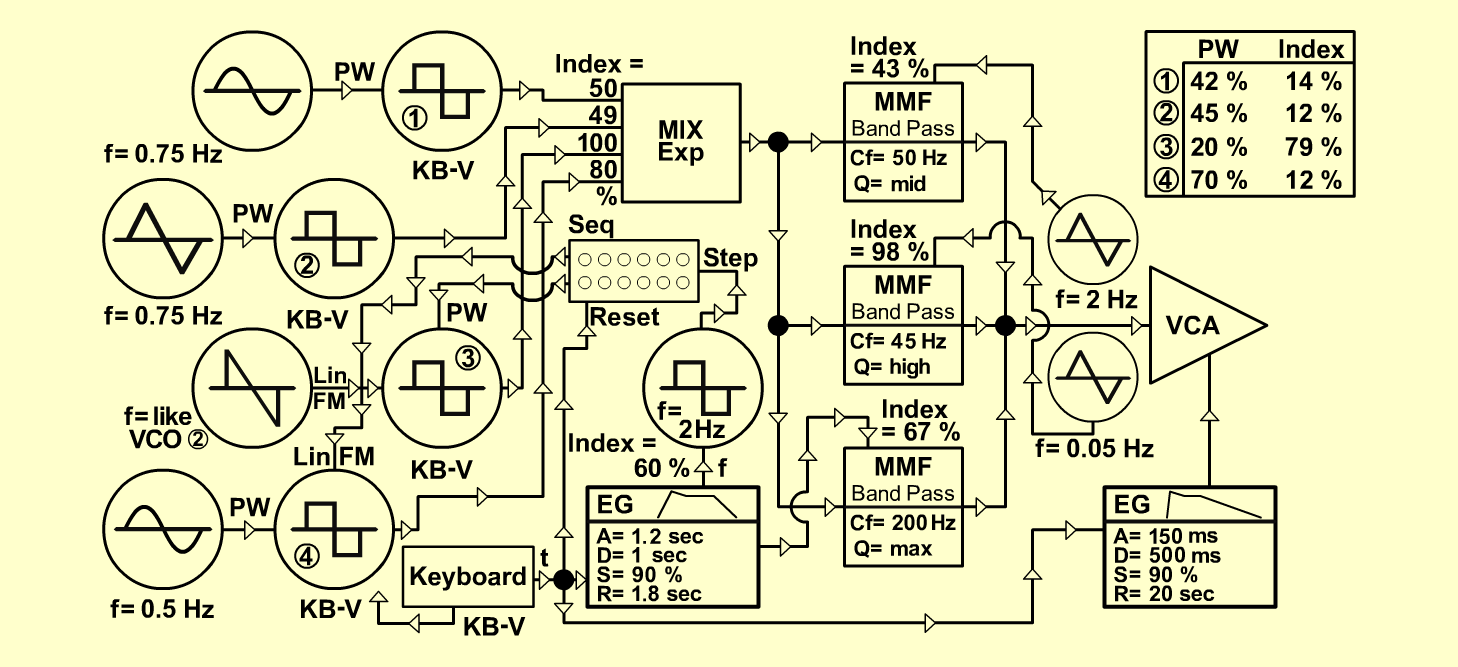PrologueTextureD o e p f e r A - 100 Connections: Settings: A-110/1 (Pulse)  <=>  A-138 (Input 1) A-110/2 (Pulse)  <=>  A-138 (Input 2) A-111/1 (Pulse)  <=>  A-138 (Input 3) A-111/2 (Pulse)  <=>  A-138 (Input 4) A-138 (Output)  <=>  A-127 (Audio In) A-127 (Mix)  <=>  A-131 (Audio In) A-131 (Audio Out)  <=> Amplifier A-145/1 (Sine)  <=>  A-111/2 (PCV) A-145/2 (Sine)  <=>  A-110/1 (PWCV2) A-145/2 (Tri)  <=>  A-110/2 (PWCV2) A-140/1 (Output)  <=>  A-131 (CV 2) A-140/2 (Output)  <=>  A-127 (Ext CV 3) A-140/2 (Output)  <=>  A-147 (CV) A-111/2 (Saw)  <=>  A-111/1 (LinFM) A-155 (Row 1: Post Out)  <=>  A-111/1 (PCV) A-155 (Row 2: Post Out)  <=>  A-111/2 (LinFM) A-147 (Pulse)  <=>  A-155 (Step/Clock) A-165 (+/- Out)  <=>  A-155 (Reset) KB-Voltage <=> A110/1, A110/2, A111/1, A111/2 (CV1) KB-Gate  <=>  A-140/1, A-140/2 (Gate) KB-Gate  <=>  A-165 (In) A-110/1 (Range = 0, Tune = 4,  PW = 4.2, PW CV 2 = 1.4) A-110/2 (Range = -1, Tune = 3.7, PW = 4.5, PW CV 2 = 1.2) A-111/1 (Lin FM = 8, Tune = 5, Fine = 5.2, PW = 2, PCV = 7.9) A-111/2 (Oct = -2, Lin FM = 10, Tune = 5, PW = 7, PCV = 1.2) A-138exp (In 1 = 5, In 2 = 4.9, In 3 = 10, In 4 = 8, Out = 10) A-131 (Gain = 0, CV2 = 10, Audio In 1 = 10,  Audio Out = 5 - 6) A-140/1 (A = 1, D = 6, S = 6, R = 6, Range = H) A-140/2 (A = 10, D = 10, S = 8, R = 10, Range = M) A-145/1 (Frq = 0.4, Range = M) A-145/2 (Frq = 1, Range = M) A-127 (Audio In = 9.1, Original = 0;  Row 1: LFO Freq = 4, CV Ampl = 4.3, VCF Frq = 2, Res = 4.2, Audio Level = 8.2;  Row 2: LFO Freq = 0.8, CV Ampl = 9.8, VCF Frq = 1.6, Res = 6.9, Audio Level = 8.2;  Row 3: LFO Freq = 4.7, CV Ampl = 6.7, VCF Frq = 3.8, Res = 9.3, Audio Level = 5.7) A-147 (Freq = 5.7, CV = 6) A-155 (Row 1: 1 = 1, 2 = 3.2, 3 = 7, 4 = 5.3, 5 = 1.8, 6 = 3.3, 7 = 6.1, 8 = 10, Range = 4V, Glide = 0; Row 2: 1 = 3.1, 2 = 4, 3 = 6, 4 = 10, 5 = 8, 6 = 1.8, 7 = 5.3, 8 = 8, Scale = 10, Glide = 8.2) Notes: The 4 vco's might need tuning to each other,or slight detuning(as you like). I played an A2 note on my keyboard, held it down some seconds, and then release... Bakis Sirros, December 2001  group: PARALLEL WORLDSSound sample Prologue